Engineering Math - Quick Reference                                 Home : www.sharetechnote.com Matrix - Determinent   To be honest, I am not sure on how to describe the mathemtical definition of a Matrix that can apply to any size of matrix. Even thought there is an algorithm (usually used in computer programming) that can calculate the determinant of a matrix with any size, in textbook you would see different method of determinant calculation is used depending on the size of the matrix. In this page, I mostly focus on the determinant of 2x2 and 3x2 matrix but the 'Practical meaning and Application of the determinant' section can be applied to any size of matrix (any size of square matrix).     Determinant of a 2x2 Matrix   As you may learned in High school math or linear algegra course, the definition of Determiant for 2 x 2 matrix as follows is very simple as shown below.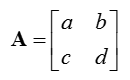Determinant of the matrix is expressed in mathmatical symbol and can be calculated as follows :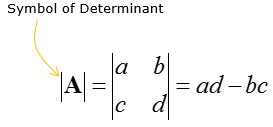But this kind of definition would not help much about understanding the real meaning of determinants and the calculation process gets exponentially complicated as the size of the matrix gets larger. If the size of matrix is equal to or greater than 4 x 4, it would be almost impossible to calculate it by hand.   So I would focus more on explaining the practical meaning of the determinants rather than calculation process.   Practical meaning of Determinant of matrix of 2 x 2 is as follows. It is the area of the shape enclosed by the two vectors which are made out of each row of the matrix.In some cases as follows, the determinants gets 0.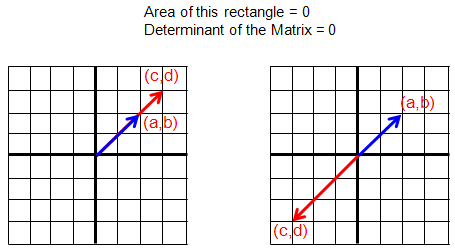Determinant of a 3x3 Matrix   Let's look into the case of 3 x 3 matrix as follows.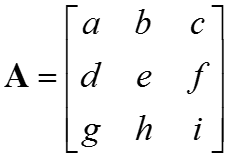Mathematical symbol for this 3x3 matrix can be represented as follows :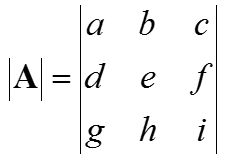How to calculate this 3x3 matrix determinant ? There are couple of ways you can find in the textbook. One of the most common way for the calcuation would be the one shown below. As you see here, rearrange the matrix as shown below (copy the first two rows and paste it at the bottom of the matrix). And group each elements as highlighted below. And then, multiply all the elements within a highlighted strap and put a negative sign for the red strap and put the positive sign for the blue strap.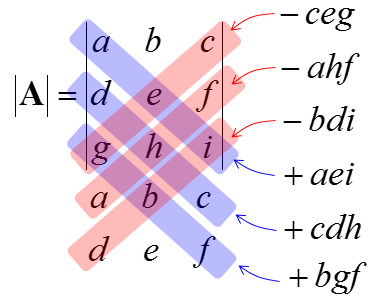Then combine all the results for each strap into a single equation. You will get the result as follows.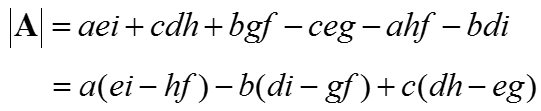Here, you can represent this result in another form as shown below. This is another commonly used method for 3x3 matrix determinant calculation. The basic idea for this method is that split a 3x3 matrix into combination of multiple 2x2 matrix determinant. As you see, 2x2 determinant calculation is very simple and repeating the several 2x2 matrix determinants calculation you can compute the 3x3 determinant.The practical meaning of Determinant of matrix is the volume of the object defined by the three vectors which is made out of each row of the matrix.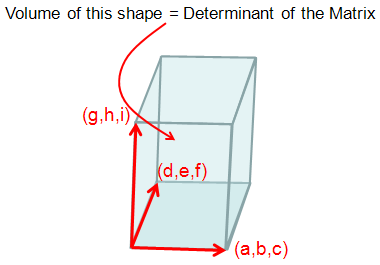If the size of matrix gets equal to/higher than 4 x 4, it would be difficult to visualize the definition geometrically as above, but I hope you can have your own image at least.     Determinant of a NxN Matrix   We have seen in previous section the method to calculate the determinant of 2x2 and 3x3 matrix ? Then, you may ask .. how can I calculate 4x4 matrix, 5x5 matrix etc ? There is no simple and easy method to do this, but the most common way would be to convert the matrix into the combinations of mutiple 2x2 or 3x3 matrix equation and then apply the method of 2x2, 3x3 determinant calculation method.     Practical Meaning and Application of the Determinant   Real importance of Determinant can be described as follows. You can understand basic characteristics of a matrix from determinant. When you apply a Matrix to vectors, you can make pretty reasonable guess just by looking at Determinant without doing all the calculation. If the process requires only one time calculation (e.g, one time multiplication of Matrix and vector) it would be no problem to perform the calculation, but if you have to do many times it would be handy to make a inference of the result from Determinant rather than doing all the 'matrix x vector' multiplication.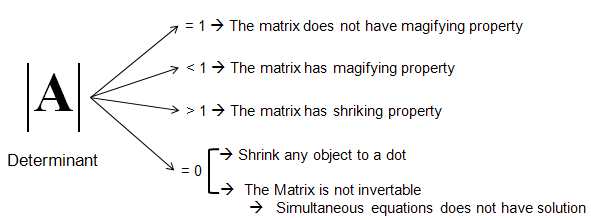You will see some examples of using the determinants of Matrix in Geometric/Graphical Meaning of Eigenvalue and Determinant